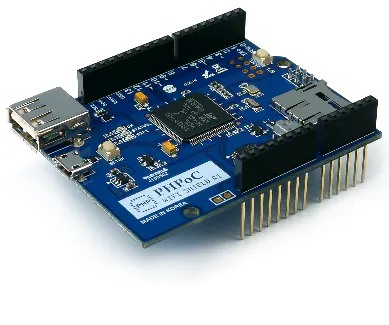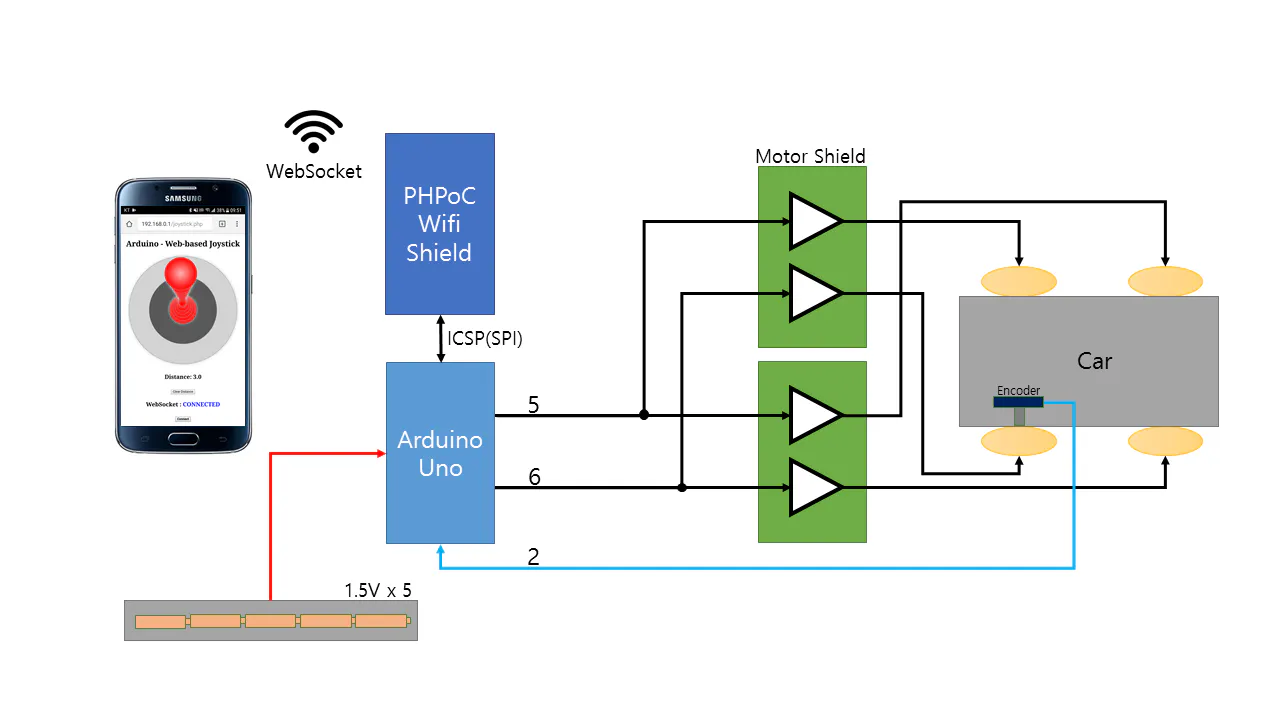# Distance Measurement Vehicle via Websocket

A vehicle measures distance with an encoder on its wheel. It is remotely controlled and transmits the distance via Websocket.

IntermediateFull instructions provided8 hours16,451

## Things used in this project

### Hardware componentsArduino UNO & Genuino UNO
×1PHPoC WiFi Shield for Arduino
×1
 DFRobot RB-Dfr-67
×1
 Rechargeable Battery AA (3050mAh)
×5
 DFRobot RB-Dfr-04
×1
 DFRobot 2x2A DC Motor Shield for Arduino
×1

## Schematics

### System Diagram

The system diagram## Code

### joystick.php

PHP
PHPoC code which controls the vehicle and monitor the current distance from the vehicle via Websocket.
I have modified it from the joystic application on hackster.io (https://www.hackster.io/iot_lover/arduino-web-based-joystick-02ca54)
```<!DOCTYPE html>
<html>
<title>Arduino - PHPoC Shield</title>
<meta name="viewport" content="width=device-width, initial-scale=0.7, maximum-scale=0.7">
<meta charset="utf-8">
<style>
body { text-align: center; font-size: width/2pt; }
h1 { font-weight: bold; font-size: width/2pt; }
h2 { font-weight: bold; font-size: width/2pt; }
button { font-weight: bold; font-size: width/2pt; }
</style>
<script>
var canvas_width = 500, canvas_height = 500;
var range = canvas_width/2 - 10;
var step = 18;
var ws;
var joystick = {x:0, y:0};
var click_state = 0;

var pos_old;

var ratio = 1;

function init()
{
var width = window.innerWidth;
var height = window.innerHeight;

if(width < height)
ratio = (width - 50) / canvas_width;
else
ratio = (height - 50) / canvas_width;

canvas_width = Math.round(canvas_width*ratio);
canvas_height = Math.round(canvas_height*ratio);
range = Math.round(range*ratio);
step = Math.round(step*ratio);

var canvas = document.getElementById("remote");
//canvas.style.backgroundColor = "#999999";
canvas.width = canvas_width;
canvas.height = canvas_height;

var ctx = canvas.getContext("2d");
ctx.translate(canvas_width/2, canvas_height/2);
ctx.lineCap="round";
ctx.lineJoin="round";

update_view();
}
function connect_onclick()
{
if(ws == null)
{
if((navigator.platform.indexOf("Win") != -1) && (ws_host_addr.charAt(0) == "["))
{
// network resource identifier to UNC path name conversion
}

ws = new WebSocket("ws://" + ws_host_addr + "/web_joystick", "text.phpoc");
document.getElementById("ws_state").innerHTML = "CONNECTING";
ws.onopen = ws_onopen;
ws.onclose = ws_onclose;
ws.onmessage = ws_onmessage;
}
else
ws.close();
}
function ws_onopen()
{
document.getElementById("ws_state").innerHTML = "<font color='blue'>CONNECTED</font>";
document.getElementById("bt_connect").innerHTML = "Disconnect";
update_view();
}
function ws_onclose()
{
document.getElementById("ws_state").innerHTML = "<font color='gray'>CLOSED</font>";
document.getElementById("bt_connect").innerHTML = "Connect";
ws.onopen = null;
ws.onclose = null;
ws.onmessage = null;
ws = null;
update_view();
}
function ws_onmessage(e_msg)
{
e_msg = e_msg || window.event; // MessageEvent
document.getElementById("distance").innerHTML = e_msg.data;
}
function send_data()
{
var x = joystick.x, y = joystick.y;
var joystick_range = range - radius_handle;
x = Math.round(x*100/joystick_range);
y = Math.round(-(y*100/joystick_range));

if(ws != null)
ws.send(x + ":" + y + "\r\n");
}
function update_view()
{
var x = joystick.x, y = joystick.y;

var canvas = document.getElementById("remote");
var ctx = canvas.getContext("2d");

ctx.clearRect(-canvas_width/2, -canvas_height/2, canvas_width, canvas_height);

ctx.lineWidth = 3;
ctx.strokeStyle="gray";
ctx.fillStyle = "LightGray";
ctx.beginPath();
ctx.arc(0, 0, range, 0, 2 * Math.PI);
ctx.stroke();
ctx.fill();

ctx.strokeStyle="black";
ctx.fillStyle = "hsl(0, 0%, 35%)";
ctx.beginPath();
ctx.arc(0, 0, radius_base, 0, 2 * Math.PI);
ctx.stroke();
ctx.fill();

ctx.strokeStyle="red";

var pre_x = pre_y = 0;
var x_end = x/5;
var y_end = y/5;
var max_count  = (radius_shaft - 10)/step;
var count = 1;

while(lineWidth >= 10)
{
var cur_x = Math.round(count * x_end / max_count);
var cur_y = Math.round(count * y_end / max_count);
console.log(cur_x);
ctx.lineWidth = lineWidth;
ctx.beginPath();
ctx.lineTo(pre_x, pre_y);
ctx.lineTo(cur_x, cur_y);
ctx.stroke();

lineWidth -= step;
pre_x = cur_x;
pre_y = cur_y;
count++;
}

var x_start = Math.round(x / 3);
var y_start = Math.round(y / 3);
lineWidth += step;

ctx.beginPath();
ctx.lineTo(pre_x, pre_y);
ctx.lineTo(x_start, y_start);
ctx.stroke();

count = 1;
pre_x = x_start;
pre_y = y_start;

{
var cur_x = Math.round(x_start + count * (x - x_start) / max_count);
var cur_y = Math.round(y_start + count * (y - y_start) / max_count);
ctx.lineWidth = lineWidth;
ctx.beginPath();
ctx.lineTo(pre_x, pre_y);
ctx.lineTo(cur_x, cur_y);
ctx.stroke();

lineWidth += step;
pre_x = cur_x;
pre_y = cur_y;
count++;
}

for(var i = 85; i >= 50; i-=5)
grd.addColorStop((85 - i)/35, "hsl(0, 100%, "+ i + "%)");

ctx.fillStyle = grd;
ctx.beginPath();
ctx.arc(x, y, radius_handle, 0, 2 * Math.PI);
ctx.fill();
}
function process_event(event)
{
var pos_x, pos_y;
if(event.offsetX)
{
pos_x = event.offsetX - canvas_width/2;
pos_y = event.offsetY - canvas_height/2;
}
else if(event.layerX)
{
pos_x = event.layerX - canvas_width/2;
pos_y = event.layerY - canvas_height/2;
}
else
{
pos_x = (Math.round(event.touches.pageX - event.touches.target.offsetLeft)) - canvas_width/2;
pos_y = (Math.round(event.touches.pageY - event.touches.target.offsetTop)) - canvas_height/2;
}

return {x:pos_x, y:pos_y}
}
function mouse_down()
{
if(ws == null)
return;

event.preventDefault();

var pos = process_event(event);
pos_old = pos;

var delta_x = pos.x - joystick.x;
var delta_y = pos.y - joystick.y;

var dist = Math.sqrt(delta_x*delta_x + delta_y*delta_y);

return;

click_state = 1;

var radius = Math.sqrt(pos.x*pos.x + pos.y*pos.y);

{
joystick = pos;
send_data();
update_view();
}
}
function mouse_up()
{
event.preventDefault();
click_state = 0;
}
function mouse_move()
{
if(ws == null)
return;

event.preventDefault();

if(!click_state)
return;

var pos = process_event(event);

var radius = Math.sqrt(pos.x*pos.x + pos.y*pos.y);

{
joystick = pos;

if((Math.abs(pos_old.x - pos.x) > 5) || (Math.abs(pos_old.y - pos.y) > 5))
{
send_data();
pos_old = pos;
}
update_view();

}
}

function clear_distance()
{
if(ws != null) ws.send("c\r\n");
}
</script>

<body>

<p>
<h1>Arduino - Web-based Joystick</h1>
</p>

<canvas id="remote"></canvas>

<h2>
<p>
Distance: <span id="distance">null</span>
</p>

<h2>
<p>
<input type="button" value="Clear Distance" id="ClickMe" onclick="clear_distance();" />
</p>

<p>
WebSocket : <span id="ws_state">null</span>
</p>
<button id="bt_connect" type="button" onclick="connect_onclick();">Connect</button>
</h2>

</body>
</html>
```

### distance_measuring_machine

Arduino
This is C source code for Arduino Uno
```#include "SPI.h"
#include "Phpoc.h"

#include "TimerOne.h"

volatile long encoder1 = 0, encoder2 = 0;
unsigned long encoder1_old = 0, encoder2_old = 0;
unsigned long uptime_old = 0;

//Arduino PWM Speed Control
int EL = 5;
int ML = 4;
int ER = 6;
int MR = 7;

int amr, aml;

float max_speed;

bool state_old = 0;
int filter_count = 0;
int filter_threshold = 10;

PhpocServer server(80);

void setup() {
Serial.begin(9600);
while(!Serial)
;

Phpoc.begin(PF_LOG_SPI | PF_LOG_NET);
//Phpoc.begin();

server.beginWebSocket("web_joystick");

Serial.println(Phpoc.localIP());

pinMode(ML, OUTPUT);
pinMode(MR, OUTPUT);
digitalWrite(ML, LOW);
digitalWrite(MR, HIGH);

pinMode(2, INPUT);
digitalWrite(2, HIGH); // pull-up

encoder1 = 0;
encoder2 = 0;
encoder1_old = 0;
encoder2_old = 0;

//attachInterrupt(digitalPinToInterrupt(2), ISR_di1, FALLING);
Timer1.initialize(100); // 0.1m second
Timer1.attachInterrupt(ISR_Timer1);
}

void loop() {
double distance1;
String str;
char ch;

// wait for a new client:
PhpocClient client = server.available();

if (client) {

if(data){
if(data.substring(0, 1) == "c")
{
Serial.println("Clearing encoder1...\r\n");
encoder1 = 0;
encoder1_old = 0;
server.write("0");
return;
}
int pos = data.indexOf(':');
long x = data.substring(0, pos).toInt();
long y = data.substring(pos+1).toInt();

if(y < 0)
{
analogWrite(EL, 0);
analogWrite(ER, 0);
return;
}
else
{
max_speed = sqrt((float)x*(float)x/10000+(float)y*(float)y/10000);
max_speed *= 255;
//Serial.print("Max Speed: ");
//Serial.println(max_speed);

//Serial.print("encoder1 = ");
//Serial.println(encoder1);
}

if(x == 0)
{
aml = (int)max_speed;
amr = (int)max_speed;
}
else if(x > 0)
{
aml = (int)max_speed;
amr = (int)(max_speed*(100-abs(x))/100);
}
else if(x < 0)
{
aml = (int)(max_speed*(100-abs(x))/100);
amr = (int)max_speed;
}

analogWrite(EL, aml);
analogWrite(ER, amr);

}
}
if(encoder1 >= (encoder1_old + 10))
{
distance1 = (double)encoder1 * 0.0103;
str = String(distance1, 2);
str.toCharArray(ch, str.length());
server.write(ch);
Serial.print(encoder1);
Serial.print(":");
Serial.println(ch);
encoder1_old = encoder1;
}

}

void ISR_Timer1()
{
bool state = 0;

if(state != state_old)
{
filter_count++;
if(filter_count > filter_threshold)
{
state_old = state;
encoder1++;
}
}
if(state == state_old && filter_count > 0)
{
filter_count--;
}
}

/*
void ISR_di1()
{
unsigned long uptime_now;
uptime_now = mills();
if(uptime
encoder1++;
}
*/
```

## Credits

### Matthew Lee

2 projects • 24 followers
Hardware Engineer